The SEIR Model

• The standard model for the spread of a virus is the Susceptible, Exposed (infected, but not yet infectious), Infectious (now can infect others), Removed (SEIR) model.

• This is a system of nonlinear Ordinary Differential Equations (ODEs), which must be solved numerically.

• You can download the Matlab files main_corona.m and seir.m, and try running this yourself. The most important parameter is R_zero as a function of time.

• If you don't have access to Matlab, this code also runs under the open source Octave package .

• Here a link to the Python version (thanks to Kees de Graaf).

• Here is a link to the R version (thanks to Pierre Chausse).

• Wuhan Data

• Using parameters from the literature, the model shows that the peak number of cases in the Wuhan area should be about 140,000 by the end of March, 2020. Current data shows a much smaller number of cases. This could be due to (i) very successful efforts to control spreading (i.e. reduction of "R_zero"), (ii) undereporting of cases, or (iii) incorrect data for the SEIR model.

• The important input is Rzero vs time. Rzero is the average number of people infected by a single infectious person. Rzero greater than 1, means that infection will spread until herd immunity reached. Rzero less than 1, means that infection will die out, without infecting a large proportion of susceptible population.

• How do you change Rzero? It appears that the corona virus (without any mitigation) has an Rzero somewhere between 2.0-3.0. See paper in Cell Discovery (2020) February. Social distancing and quarantines can reduce Rzero to less than one.

•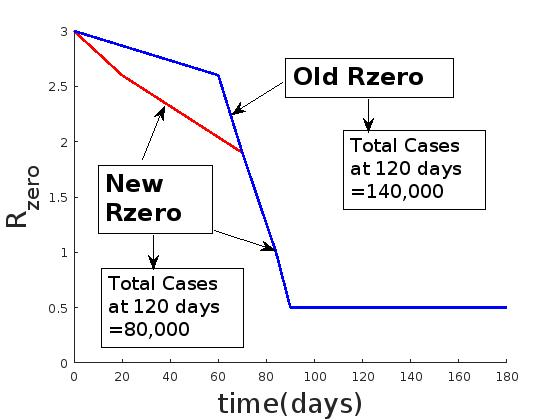• Made small change in R_zero vs. time, this has a very large effect. Now results are closer to observed data in Wuhan. This indicates that these models are very sensitive to this data. Total active cases now peaks at around 90 days. Cumulative total cases at 120 days is about 80,000.

• The bad news. The population of Wuhan is about 11 million. After 180 days, at most 100,000-200,000 will have been infected, recovered, and are now immune. This hardly makes a dent in the susceptible population. This means that any new outbreak will start the process all over again. Can economies stand several waves of social distancing mitigation?
•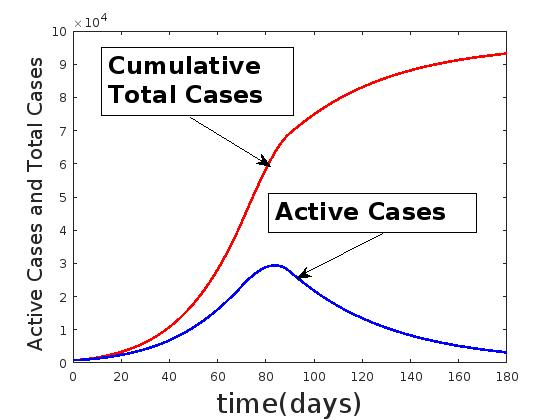The Next Wave

Suppose that we assume the data from Wuhan. Now, suppose that social distancing is relaxed beginning at 120 days. A gradual lifting of mitigation occurs between 120 days and 240 days, so that at 240 days, Rzero is now 1.75. As we shall see, this causes a second wave of infection, so that once again, social distancing is imposed, at day 240, with Rzero returning to 0.5 at day 360. The second wave actually peaks higher than the first wave. This assumes that the beginning of the next wave is recognized early, but the reintroduction of social distancing takes longer the second time around (i.e. more people need to keep working).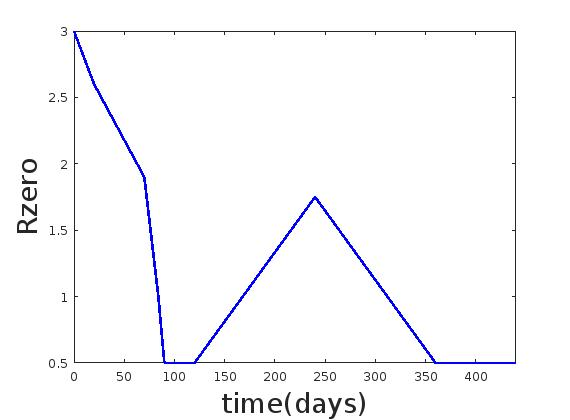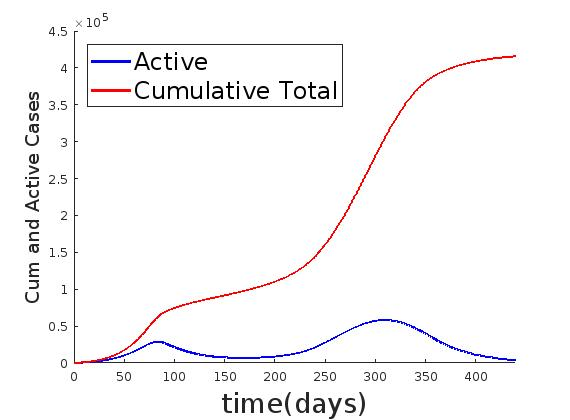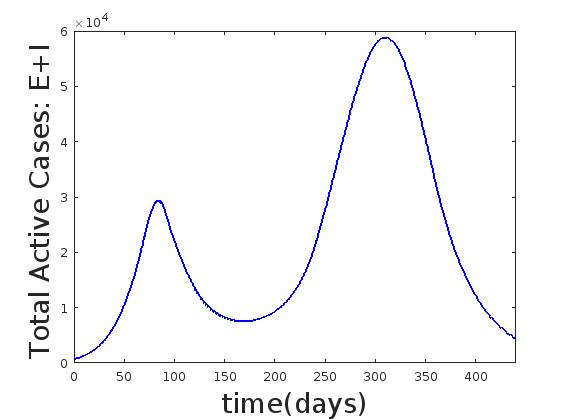All Models are Wrong, Some are Useful

This is a quote from the statistican George Box. There has been much criticism in recent days about model predictions, and the widely varying forecasts for the number of coronavirus cases. As you can see from the above, small changes in the R_zero input have a large effect on the output. With no social mitigation efforts, this number is already hard to determine. Now, you also have to determine how social distancing and quarantines effects R_zero, and how fast this occurs. You should try different scenarios for R_zero yourself, to get a feel for how difficult it will be to make reliable forecasts.

But, as Box points out, it is useful to play around with these models, since it gives you an understanding of the factors at play.

Research Question

The SEIR model assumes a "well mixed" homogeneous population. This is obviously not true over a large geographic region. One way to extend this model is to assume that (S,E,I,R) are spatially distributed. Then, the quantities which appear on the rhs of the ODE system are spatially smoothed. This will give rise to a system of Partial Integro-Differential Equations (PIDEs). See Archiv paper arXiv:1909.01330. Another approach is to use a network based SEIR model, i.e. nodes in the network represent different populations. Contacts between the populations occur through a network See Archiv paper arXiv:2006.06939. In addition, there is a large amount of randomness associated with disease spread, so a better model uses stochastic differential equations to simulate pandemics.

The GLEAM model includes both spatial and stochastic effects.

Webinar April 29, 2020 at 2:30pm (GMT+1)

Mathematics of the COVID19 crisis – In the eye of the storm